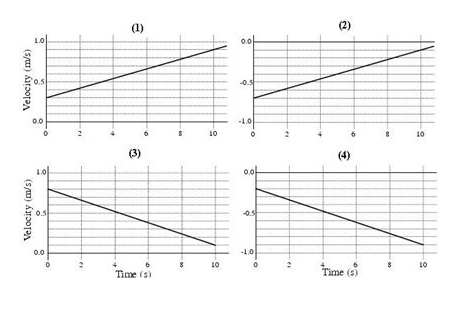# Problem: (a) Which graphs represent an object moving in the negative direction?(b) Which graphs represent an object that is speeding up?(c) Which graphs represent an object that has a negative acceleration?

###### FREE Expert Solution

(a) An object moves in the negative direction if the velocity is negative. Velocity is negative for graphs 2 and 4. Do NOT confuse this with the SLOPE of the velocity graphs, which is negative for Graphs 3 & 4.

Graphs 2 and 4 represent an object moving in the negative direction.

88% (43 ratings)###### Problem Details(a) Which graphs represent an object moving in the negative direction?

(b) Which graphs represent an object that is speeding up?

(c) Which graphs represent an object that has a negative acceleration?